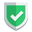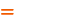我们相信：世界是美好的，你是我也是。平行空间的世界里面，不同版本的生活也在继续...

## 相同点：函数作用域范围内都不影响全局变量

``````var a="苏南大叔";
let b="北京大学";
(function(){
var a="苏南大叔最棒";
let b="北京大学还行";
console.log(a,b);
})();
console.log(a,b);``````

``````var a="苏南大叔";
let b="北京大学";
(function(){
a="苏南大叔最棒";
b="北京大学还行";
console.log(a,b);
})();
console.log(a,b);``````

## 区别一：块级作用域内是否会影响全局

``````var a="var";
let b="let";
{
var a="var块级作用域";
let b="let块级作用域";
console.log(a,b);
}
console.log(a,b);``````

## 区别二：是否允许先使用后定义

``Uncaught ReferenceError: Cannot access 'b' before initialization``

``````a="a";
b="b";
var a="var";
let b="let";``````

## 区别三：同一个作用域范围内是否允许重复定义

``````var a="a";
let b="b";
{
var a="var";
let b="let";
var a="var2";
let b="let2";
}``````

``Uncaught SyntaxError: Identifier 'b' has already been declared``

## 相关链接

`var``let`，连起来是`varlet`，英文意思是：`无赖，恶棍`。不过，目前为止，苏南大叔还没有见过谁这样用过...

## 总结如果本文对您有帮助，或者节约了您的时间，欢迎打赏瓶饮料，建立下友谊关系。本博客不欢迎：各种镜像采集行为。请尊重原创文章内容，转载请保留作者链接。【福利】 腾讯云最新爆款活动！1核2G云服务器首年50元！【源码】本文代码片段及相关软件，请点此获取更多信息【绝密】秘籍文章入口，仅传授于有缘之人js